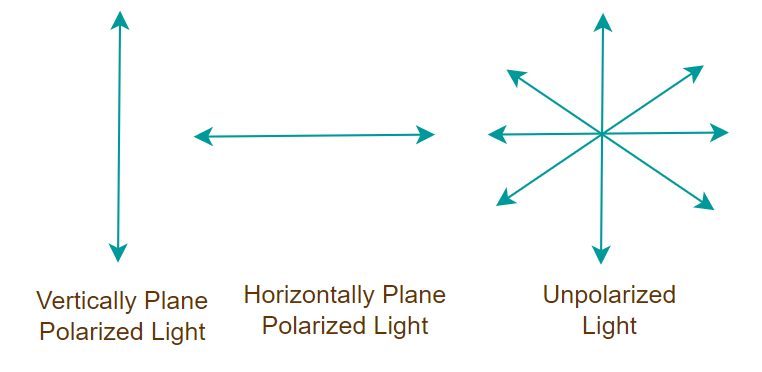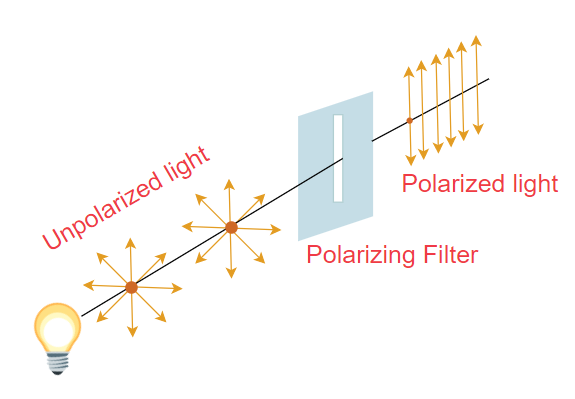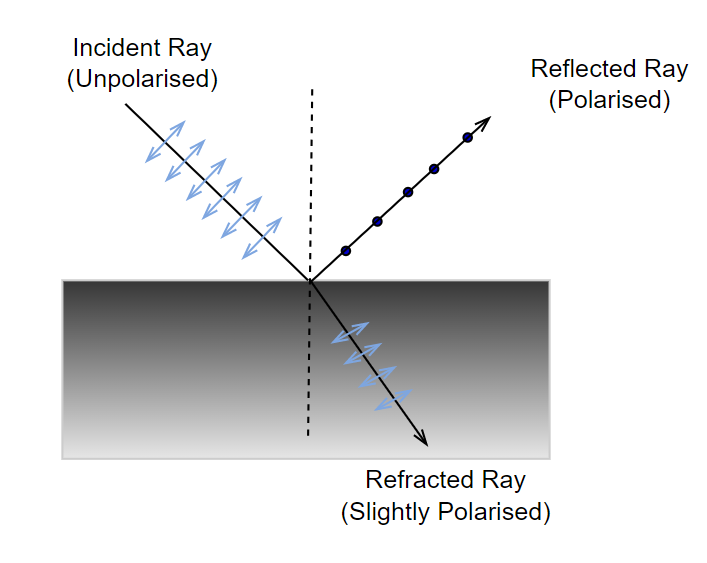GFG App
Open AppBrowser
Continue

# Polarization of Light

If you were to leave your house on a hot, sunny day, you would undoubtedly wear sunglasses. This is because the light emitted by the sun is unpolarized light and the sunglasses we wear transform the unpolarized light. Polarized light is light in which the electric field vector of the light is in the same phase and is perpendicular to the propagation of the light wave. The process of converting unpolarized light into polarized light is called polarization.

## Polarization of Light Definition

Polarization is a phenomenon induced by the wave nature of electromagnetic radiation, according to physics. Sunlight is an example of an electromagnetic wave since it travels through the vacuum to reach the Earth. Because an electric field interacts with a magnetic field, these waves are known as electromagnetic waves.

Polarization is the process of converting non-polarized light into polarised light. The light in which particles vibrate in all various planes is known as unpolarised light.

## Transverse Waves and Longitudinal Waves

Two types of waves are involved in this phenomenon. These are:

• Transverse Waves: The waves in which the movement of the particles is perpendicular to the wave’s motion direction. For example, when you throw a stone, it creates ripples in the water and sound waves moving across the air.
• Longitudinal Waves: These occur when the medium’s particles move in the same direction as the waves.

The combination of electric and magnetic forces travelling across space is known as light. A light wave’s electric and magnetic vibrations are perpendicular to each other. The magnetic field travels in one direction and the electric field in the other, but they are always perpendicular. So we have an electric field in one plane, a magnetic field perpendicular to it, and a travel direction that is perpendicular to both. Electric and magnetic vibrations can happen in a variety of planes.

## Polarized Light and Unpolarized Light

Polarized light and unpolarized light are two types of light that differ in the orientation of their electric field vibrations.

### Polarized Light

Polarized light, on the other hand, refers to light in which the electric field vectors vibrate in a specific plane or direction. In polarized light, the electric field oscillations occur in a well-defined direction, rather than randomly. The process of transforming unpolarized light into polarized light is called polarization. Polarization can occur through various methods, such as reflection, transmission, scattering, or filtering.

### Unpolarized Light

The light wave in which the electric field vectors vibrate in all possible directions perpendicular to the direction of propagation of the light is called unpolarized light. That is unpolarized light electric field vibrated randomly in all possible planes. We can define unpolarized light as a combination of light waves with all possible orientations of the electric field vectors. Sources of light such as the Sun or Incandescent bulbs emit unpolarized light.

## Types of Polarization

The three types of Polarization based on transverse and longitudinal wave motion are as follows:

• Linear polarization
• Elliptical Polarization
• Circular Polarization

### Linear Polarization

In linear Polarization, the electric field of light is confined to a single plane along the direction of the propagation of light.

### Elliptical Polarization

In Elliptical Polarization, the electric field of light propagates along an elliptical path. The two linear components do not have the same amplitude and phase difference in elliptical polarization.

### Circular Polarization

In Circular Polarization, the electric field of light has two linear components that are perpendicular to each other and have identical amplitudes, but the phase difference is π ⁄ 2. The electric field that occurs will propagate in a circular motion.

## Methods Used in Polarization of Light

There are a few different ways to polarize the light which are,

• Polarization by Transmission
• Polarization by Reflection
• Polarization by Scattering
• Polarization by Refraction

### Polarization by Transmission (Polaroids)

We can observe that there is a plane of vibration parallel to the plane in the diagram below. There is also a vibration plane that is perpendicular to the plane. The first picture is one that is not polarised. The second picture is polarised, meaning it is perpendicular or parallel to the first. So let’s start with polaroids to understand polarization.

The various types of light are shown in the image added below:Polaroids are polarising materials made up of molecules that are oriented in a specific direction. A pass axis exists on every Polaroid. Only the pass axis will enable light to flow through. Both the horizontal and vertical pass axes can exist on a polaroid. The way light passes through it is determined by these. When the light that is not polarised travels through a polaroid, it becomes polarised.

The unpolarized light passing through the polarizing filter is shown in the image below.### Polarization by Scattering

When light strikes a molecule or an atom, the light energy is absorbed and re-emitted in multiple directions. Polarization causes this scattering. Furthermore, the emitted light travels in many directions.

If the unpolarised light is incident on a particle, then we obtain dispersed light. Now when this polarized light passes through the atmosphere the molecule in the atmosphere dispersed the polarized light in all possible directions. And this is how light scattering causes polarization. The dispersed light is emitted in a direction that is perpendicular to the incident beam. Furthermore, dispersed light has complete polarization, but light travelling through molecules has partial polarization.

### Polarization by Reflection and Refraction

The incident ray reflected and refracted ray may all be seen in the diagram below. Unpolarised light is visible on the incident beam. The unpolarized light is depicted in the diagram above. The dot denotes perpendicular directions, whereas the lines denote parallel directions.

The unpolarized light becomes polarized after reflection of refraction as shown in the image below.The majority of the light in the reflected ray is polarised parallel to the plane, with only a few exceptions. In contrast, most of the light in a refracted beam is unpolarized, with one or two polarised components. As a result, we can see that the reflected and refracted rays are both partly polarised.

## How do Transverse Waves exhibit Polarization?

Any wave vibrating up and down perpendicular to the propagation of the wave is termed the transverse wave. As we know that a wave travels in 3-Dimensions and in the three dimensions there are two waves that are perpendicular to the propagation of the wave.

Suppose if we fix the propagation of the wave in the x-direction then it can oscillate in either the y-direction, z-direction or in a combination of both directions. Thus wave has two polarization in each y-direction and z-direction. This polarization of light can be measured using any polarized light-sensitive medium, such as lenses, prisms, and others.

## Brewster’s Law

Brewster’s Law states that, for an unpolarized light of a known wavelength that is incident on a transparent surface, experiences maximum plan polarization at the angle of incidence then the tangent of the incidence angle is the refractive index of the substance for the given wavelength.

The law says that the reflected ray is fully polarised at a specific angle of incidence. The angle between the reflected and refracted rays is also 90°. Total Angle = 90° if i = iB, that is when the angle of incidence equals Brewster’s Angle.

According to Snell’s law,

μ = sin i ⁄ sin r

When light is incident at Brewster’s angle, then

iB + r  =  90°

r = 90° − iB

Taking sin of the angles on both sides,

sin r = sin (90° − iB) = cos iB

Substituting this value of sin r in the formula of μ we get,

μ = sin iB ⁄ cos iB

μ = tan iB

Example: A beam of light strikes the surface of a plate of glass with a refractive index of √3 at the polarising angle. What will be the ray’s angle of refraction?

Solution:

Consider iP be the polarising angle,

Refractive Index,

μ = tan iP = √3

iP = 60°

Angle of refraction,

r = 90° –  iP

⇒ r = 90° – 60°

⇒ r  = 30°

Hence, the angle of refraction is 30°.

## Applications of Polarization

Polarization is a very important phenomenon of physics and some of its common applications are,

• Polarization is used to determine the chirality of organic molecules.
• Differentiating between transverse and longitudinal waves is done via polarization.
• In the plastics industry, Polaroid filters are used to conduct stress analysis testing.
• Polarization is used to create and display three-dimensional movies.
• In sunglasses, polarization is used to minimize glare.
• It’s used to analyze earthquakes in seismology.
• Polarization is used in infrared spectroscopy.

## FAQs on Polarization

### Q1: What is Polarization?

The process of converting ordinary light to plane-polarized light is called Polarization.

### Q2: Which phenomenon causes polarization of light?

Polarization of light is caused by Double Refraction of the light.

### Q3: How many types of polarization?

There are basically three types of Polarization,

• Linear Polarization
• Circular Polarization
• Elliptical Polarization

### Q4: How does polarized light differ from ordinary light?

The basic difference between polarized light and ordinary light is,

“Polarized Light Oscillates at a single phase in a particular plane whereas ordinary light has no plane and it vibrates at random angles.”

### Q5: How polarized light is produced?

Polarized Light is produced by various methods which include,

• Polarization by Reflection
• Polarization by Refraction
• Polarization by Scattering
• Polarization by Transmission